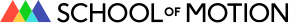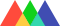#School of Motion## How to Use the Time Expression in After Effects

• By Ryan Plummer
• Share

## Ready to unlock a new skill? Let's take a look at how to use the time expression in After Effects.

One of my favorite expressions in After Effects is the time expression. While you may think you know how to use the time expression in After Effects, I've found that there's more to this simple chunk of code than meets the eye.
If you've never been introduced to the time expression then this article was designed for you. In this post I'll break down what it's doing and how After Effects interprets this small piece of code. So let's cut the chatter and get going!

## What is the Time Expression in After Effects?

I'm the type of person who picks books with a lot of pictures in them, so I'd like to start off this article with a GIF!
The time expression in After Effects returns a composition's current time in seconds. You can write the time expression in After Effects by simply typing the word time;
`time;`
The values generated by this expression can then be used to drive movement by connecting a property value to the expression.
In the example above I rigged a text layer to preview the value generated by the time expression. As the composition is playing you see the seconds being counted in the composition panel through that rigged text layer. All I did was used a simple time expression to have After Effects generate those values.
`time.toFixed(2);`
Note: toFixed() limits how many numbers are allowed after the decimal

## How Does the Time Expression Work in After Effects?

To help illustrate explain exactly what I mean, I want you to think about time in a new way. Try to think of time as the number it's producing and not as a time counter. When you can start to view time as a number that can be manipulated then you will start getting a better grasp on this expression.
For example, If I double the time expression using multiplication it would read 8 seconds within a 4 second composition time.
`time*2;`
To further drive it home i'll add the time expression to the rotation property. The rotation property will return 1 degree per 1 second.
For every second the composition runs the rotation will increase by one degree. But, that example is kinda boring and you may not be able to see much change very well. Let's speed things up a little!
Just look at that little line go! In the first example we get 1 degree for every second. So if we want to get a full rotation every second we need to know how many degrees are in 1 full rotation; which is 360 degrees.
`time*360;`
By multiplying the value time provides by 360 we are asking After Effects to speed up the process exponentially. It now is going to complete a 1 degree move 360 times within one second.

## Cool Examples of the Time Expression in After Effects

Now that you've wrapped your head around what time is doing, let's show you some practical examples you can start using in your workflow.
Rotate Multiple Layers
Here is an example of looping rotations at different speeds. Imagine if you had a bunch of gears that needed to rotate, or an astroid field that needed slight rotations for those cold heavy rocks.
I took the time expression and multiplied them by different amounts! As a bonus, I'd like to share a neat trick that I first learned from Parker Young's expression courses on Animoplex.
For rotation, multiply time by 360, which is one full rotation, and then divide it by the number of seconds you would like a full rotation to happen. Here's what that would look like in code:
```// One full rotation every 2 seconds
time*(360/2);```
Time Travel, Sorta...
One really useful way to harness the time expression is creating delayed movements. We can actually ask After Effects to look forwards and backwards in time. For this I'm going to introduce a new expression valueAtTime();.
For this example I asked After Effects to look at the x position of another layer, and then told it to delay by half a second. Amazingly, the code is super simple, and by using the index of a layer you can duplicate over and over again with each layer having it's own delaying. Note: The index expression in After Effects pulls a value based on the layer's order in the timeline.
`thisComp.layer(index+1).transform.xPosition.valueAtTime(time - .5)`
Does that expression seem confusing? Zack Lovatt is a fan of breaking down the various parts of code into common language so it's easier to understand. Here is how he would break down valueAtTime:
```var halfASecond = 0.5;
var now = time;
var halfASecondAgo = now - halfASecond;valueAtTime(halfASecondAgo);```
In a nutshell, valueAtTime is an expression that tells After Effects to pull a value from a property (scale, position, slider, etc.) for a declared time.

### Make it Rain!

If you want to try out something fun, I'm handing off a simple project file to you. Inside you'll find a money counting rig tied to time. I've placed a slider effect in there that allows you to increase how fast the money value is increasing! If you'd like to know how I added the dollar sign to the money counter, I've left some notes in my expression.